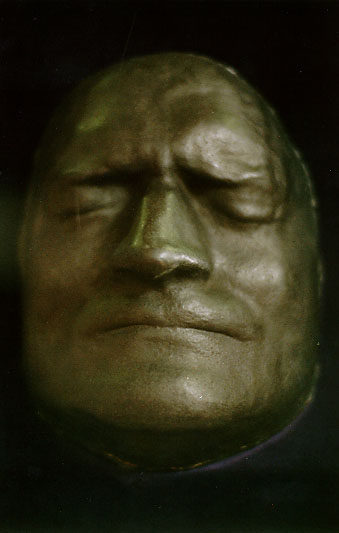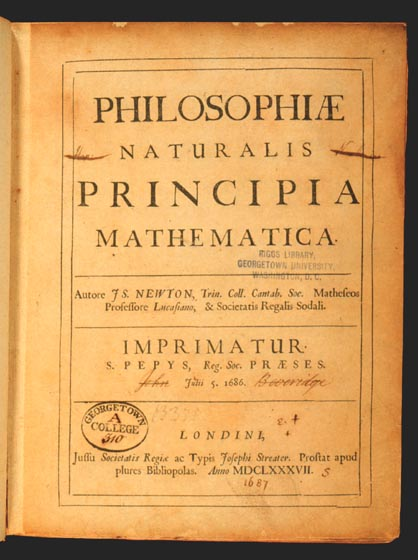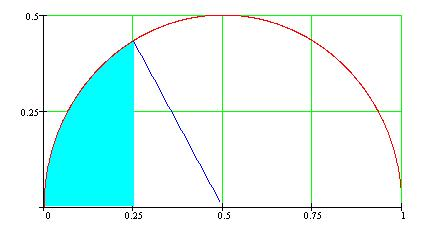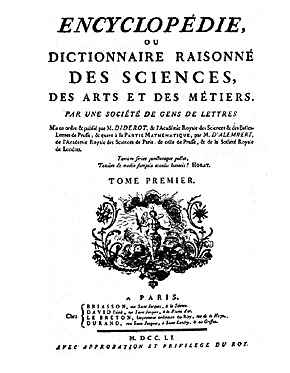U n i v e r s i t é  Y O R K   U n i v e r s i t y ATKINSON FACULTY OF LIBERAL AND PROFESSIONAL STUDIES SCHOOL OF ANALYTIC STUDIES & INFORMATION TECHNOLOGY S C I E N C E   A N D   T E C H N O L O G Y   S T U D I E S   STS 3700B 6.0 HISTORY  OF  COMPUTING  AND   INFORMATION  TECHNOLOGY   Lecture 11:  Newton and the Beginning of the Modern Era | 0 | 1 | 2 | 3 | 4 | 5 | 6 | 7 | 8 | 9 | 10 | 11 | 12 | 13 | 14 | 15 | 16 | 17 | 18 | 19 | 20 | 21 | 22 |   Topics "Nature is pleased with simplicity, and affects not the pomp of superfluous causes." "If I had staid for other people to make my tools and other things for me, I had never made anything of it."                                                                              Isaac NewtonNewtons's Deathmask(Isaac Newton Institute for Mathematical Sciences)   As far as science is concerned, the figure of Isaac Newton (1643 - 1727) does stand as a watershed between the ancient world and the modern one. The introduction of the so-called "scientific method" is often attributed to Newton. Even though such a claim is very questionable, it suggests the fundamental role that he played in transforming physical science into an experimentally grounded, yet analytical, quantitative, mathematical enterprise (consider, for example, the very title of his fundamental work, Philosophiae Naturalis Principia Mathematica). It is not possible to do justice to Newton's contributions in mathematics, optics, physics and astronomy in such a brief space, also because to appreciate his work one needs a considerable background in mathematics and its history—a prerequisite which is not mandatory in this course. We will limit ourselves, therefore, to short remarks about Newton's major accomplishments, and focus in some detail on his computational work. "… he laid the foundations for differential and integral calculus, several years before its independent discovery by Leibniz. The 'method of fluxions', as he termed it, was based on his crucial insight that the integration of a function is merely the inverse procedure to differentiating it. Taking differentiation as the basic operation, Newton produced simple analytical methods that unified many separate techniques previously developed to solve apparently unrelated problems such as finding areas, tangents, the lengths of curves and the maxima and minima of functions. Newton's De Methodis Serierum et Fluxionum was written in 1671 but Newton failed to get it published and it did not appear in print until John Colson produced an English translation in 1736." [ from op. cit. ] In 1669, when he was only 27, Newton was appointed Lucasian Professor at the University of Cambridge. There he worked on and taught optics. One of his first important discovery was that sunlight (or white light) is not a simple entity, as it had been commonly assumed since at least Aristotle. In a series of clever and simple experiments Newton showed that white light is actually a mixture of different color which, when traversing a refractive medium, separate due to their different indices of refraction. "The Principia is recognised as the greatest scientific book ever written. Newton analysed the motion of bodies in resisting and non-resisting media under the action of centripetal forces. The results were applied to orbiting bodies, projectiles, pendulums, and free-fall near the Earth. He further demonstrated that the planets were attracted toward the Sun by a force varying as the inverse square of the distance and generalised that all heavenly bodies mutually attract one another. Further generalisation led Newton to the law of universal gravitation: '…all matter attracts all other matter with a force proportional to the product of their masses and inversely proportional to the square of the distance between them.' Newton explained a wide range of previously unrelated phenomena: the eccentric orbits of comets, the tides and their variations, the precession of the Earth's axis, and motion of the Moon as perturbed by the gravity of the Sun. This work made Newton an international leader in scientific research." [ from op. cit. ]The Front Page of the First Edition of the Principia(Georgetwon University Library)   Perhaps an easy way to show the place of Newton in the development of computational mathematics is to browse through Erik Meijering's A Chronology of Interpolation: From Ancient Astronomy to Modern Signal and Image Processing. As quoted in this article, in 1665 Newton had already identified and solved one of the crucial problems encountered when one tries to fit a geometrical curve to a set of experiemntal data: "I have another method not yet published by which the problem is easily dealt with. It is based upon a convenient, ready and general solution of this problem. To describe a geometrical curve which shall pass through any given points. [ … ] Although it may seem to be intractable at first sight, it is nevertheless quite the contrary. Perhaps indeed it is one of the prettiest problems that I can ever hope to solve." Meijering's article, although rather technical, makes it apparent that Newton's work forms a core out of which much modern computational methods originated. Notice, in particular, the reference to Shannon, which we will consider later in the course. Another fundamental computational discovery is what is known today as the Newton-Raphson Theorem. This result was independently arrived at by Netwon and Joseph Raphson (1648 - 1715). In fact the two exchanged much correspondence, and they must share the credit for this discovery. The basic idea is best illustrated by an example that Newton himself uses: to find the root(s) of x3 - 2x - 5 = 0 lying between, say, 2 and 3. A modern way to deduce the answer can be found, for example, in a Module for Newton's Method designed by John Mathews, at CSUF, where you can also find further references, and glimpse the role which such a discovery had in subsequent computational mathematics. For completeness sake, here is a somewhat simplified statement of the theorem: Assume that f is a continuous function over the interval [a,b], and that there exists a number p in that interval such that f(p) = 0. If f'(p) is not 0, then there exists a δ > 0 such that the sequence {pk} defined by the iteration pk+1 = pk - f(pk)/f'(pk) for k = 0, 1, … will converge to p for any initial approximation p0 ε [p - δ, p + δ]. I will finish this sampling of Newton's contribution to computational mathematics by mentioning Newton's technique for calculating π. Newton considers a circle of radius 1/2, and calculates the area of the blue sectorNewton's Method for Calculating π (1671)   Using a variety of powerful techniques he had himself devised in developing the calculus, Newton finds that the area is equal to π/24 - √3/32, from which π = 3.1415926536, with an error of only 0.00001476. This result looks impressive only if we consider that previously a similar result would have required pages and pages of tedious calculations. You can see how quickly Newton reached his result in How Newton Calculated p. What else could we say about computational mathematics in the eighteenth century? Quite a lot! See, for example, thetimeline at MacTutor. This century saw giants such as Bayes, the Bernoulli's, Condorcet, D'Alembert, Gauss, Lagrange, Laplace. Legendre, Leibniz, Taylor, etc., who advanced mathematics by leaps and bounds and applied it not only to the physical sciences, but also to the social sciences, from the theory of probabilities to actuarian tables. Browse through the MacTutor biographies. I will therefore mention only a handful of names, beginning with Jean Le Rond d'Alembert (1717 - 1783). "D'Alembert helped to resolve the controversy in mathematical physics over the conservation of kinetic energy by improving Newton's definition of force in his Traité de Dynamique which he published in 1743." "…d'Alembert was a mathematician, not a physicist, and he believed mechanics was just as much a part of mathematics as geometry or algebra. Rational mechanics was a science based on simple necessary principles from which all particular phenomenon could be deduced by rigorous mathematical methods…d'Alembert thought mechanics should be made into a completely rationalistic mathematical system." [ M Muller, as quoted in op. cit. ] In this regard it is interesting to note that "D'Alembert stated his position clearly that he believed mechanics to be based on metaphysical principles and not on experimental evidence. He seems not to have realised in his reading of Newton's Principia how strongly Newton based his laws of motion on experimental evidence. For d'Alembert these laws of motion were logical necessities." [from op. cit. D'Alembert was also "a pioneer in the study of partial differential equations" and its applications in physics, and his Traité de Dynamique, dans lequel les Loix de l'Équilibre & du Mouvement des Corps sont réduites au plus petit nombre possible, & démonstrées d'une maniére nouvelle, & où l'on donne un Principe général pour trouver le Mouvement de plusieurs Corps qui agissent les uns sur les autres, d'une manière quelconque (1743) is indeed a "'landmark in the history of mechanics', containing the enunciation of what is now known as d'Alembert's principle. This is the method of reducing the laws of the motion of bodies to situations of static equilibrium as a technique for dynamic analysis ('the internal forces of inertia must be equal and opposite to the forces that produce the acceleration'). It is based on the three laws of motion that d'Alembert presents earlier in this work, the law of inertia, the parallelogram of motion, and the law of equilibrium and the conservation of momentum; 'he actually assumed the conservation of momentum and defined mass accordingly. This fact was what made his work a mathematical physics rather than simply mathematics' (DSB). This work was the foundation for Lagrange's classic book on analytical mechanics which codified the laws governing the motions of any systems of bodies. D'Alembert is also credited with laying to rest, in the Traité, the vis viva controversy by investigating its philosophical basis and dismissing its ontological reality. 'In this way d'Alembert was clearly a precursor of positivistic science.'" [ from D'Alembert's Principle ] At least outside the scientific circles, however, D'Alembert became famous for authoring, with Denis Diderot (1713 - 1784), the Encyclopédie, ou Dictionnaire Raisonné des Sciences, des Métiers et des Arts (1751), the grat masterpiece of the French enlightenment. This was a huge project. D'Alembert, who was initially supposed to cover only mathematics and physical astronomy, ended up compiling a much borader set of entries. The Encyclopédie included a Preface by D'Alembert, which is in itself a great work. You can read a brief excerpt in Jean Le Rond d'Alembert: Preliminary Discourse to the Encyclopedia of Diderot.The Front Page of the Encyclopédie   The other figure which we must mention is Leonhard Euler (1707 - 1783). Euler was a true 'Renaissance' man. Here is a concise summary of some of the areas he contributed to: … cartography, science education, magnetism, fire engines, machines, and ship building… The core of his research program was now set in place: number theory; infinitary analysis including its emerging branches, differential equations and the calculus of variations; and rational mechanics. He viewed these three fields as intimately interconnected. Studies of number theory were vital to the foundations of calculus, and special functions and differential equations were essential to rational mechanics, which supplied concrete problems." [ quoted in op. cit. ] "Euler's work in mathematics is so vast that an article of this nature cannot but give a very superficial account of it. He was the most prolific writer of mathematics of all time." In 1765 he published Theoria Motus Corporum Solidorum in which he showed that the motion of any solid body can be thought of as consisting of the combination of a purely rectilinear motion and a rotational motion. In conclusion, it should be mentioned that Euler's Mechanica (1736-37), was the first successful attempt to present Newtonian physics in rigorous mathematical form. Strangely enough, not much happened in the seventeeth century in terms of calculating machines. It seems as if this century was more concerned with developing and establishing on firm ground the mathematical machinery. The search for new hardware was to resume in the next century.   Readings, Resources and QuestionsRead one of three short but good biographies of Newton. The first was written by Bernard le Bovier de Fontenelle just one year after Newton's death. The second focuses more directly on the scientific aspects of Newton's work, and is a chapter from W W Rouse Ball, A Short Account of the History of Mathematics (4th edition, 1908). The third is by Robert Wilson.Visit the Museum of the History of Science, which is currently featuring The Geometry of War: 1500 - 1750. Can you find evidence that computing is a component of such geometry? Read up on some of the great protagonists we had to neglect in this lecture. Although I usually cover it in the in-class lectures, I urge you to consider the broader context of our path through the history of computing. What was happening in the arts, in literature, in politics, in economics, etc. in the various periods we have been examining? A useful place to start is AlternaTime: A Collection of Timelines on the Web.   © Copyright Luigi M Bianchi 2001, 2002, 2003 Picture Credits: Isaac Newton Institute for Mathematical Sciences Georgetown U Library · The Art Bin Last Modification Date: 11 April 2003